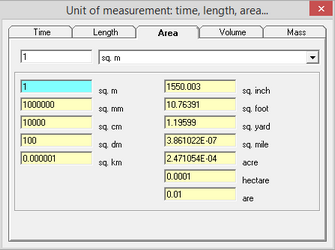﻿ Help > Unit converter (Part 2) > Area

# Area

Navigation:  Help > Unit converter (Part 2) >

# AreaTab "Area" of the dialog window "Unit converter (part 2)"

 Acre (ac) The acre is a unit of land area used in the imperial and US customary systems. It is traditionally defined as the area of one chain by one furlong (66 by 660 feet), which is exactly equal to 10 square chains, ​1⁄640 of a square mile, or 43,560 square feet, and approximately 4,047 m2, or about 40% of a hectare. Based upon the International yard and pound agreement of 1959, an acre may be declared as exactly 4,046.8564224 square metres. Hectare (hа) The hectare is a non-SI metric unit of area equal to a square with 100-metre sides, or 10,000 m2, and is primarily used in the measurement of land. There are 100 hectares in one square kilometre. An acre is about 0.405 hectare and one hectare contains about 2.47 acres. Are (а) Are, basic unit of area in the metric system, equal to 100 square metres and the equivalent of 0.0247 acre. 1 a = 100 m2 = 0.01 ha.